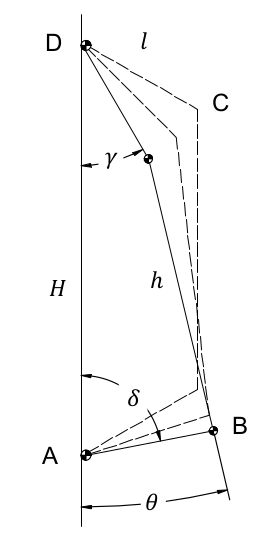# Annex A - Cinematic Analysis¶

This annex is intended to study the kinematic motion of the four-bar linkage. The final objective of this section is to find two direct relations to calculate γ and δ depending on θ with a fixed linkage geometry. Some fundamental variables are defined as follows:

$\begin{split}\begin{array}{ll} H & \mbox{the frame AD;} \\ l & \mbox{the two rockers AB e CD;} \\ h & \mbox{the coupler BC;} \\ \gamma, \delta & \mbox{the actual angles between the frame and the rockers;} \\ \theta & \mbox{the angle formed by the coupler and the frame, with a positive} \\ & \mbox{sign when the antenna points downwards.} \end{array}\end{split}$Figure A-1 The four-bar linkage

The following equations come from simple geometric considerations:

$\begin{split}\begin{array}{lr} \begin{cases} H = l \cdot \cos\gamma + h \cdot \cos\theta + l \cdot \cos\delta \\ l \cdot \sin\delta = l \cdot \sin\gamma + h \cdot \sin\theta \end{cases} & (A.1) \end{array}\end{split}$

rearranging the equations, the following can be obtained:

$\begin{split}\begin{array}{lr} \begin{cases} l \cdot (\cos\gamma + \cos\delta) = H - h \cdot \cos\theta \\ l \cdot (\sin\gamma - \sin\delta) = h \cdot \sin\theta \end{cases} & (A.2) \end{array}\end{split}$

extracting l and replacing it in the first equation, it results as:

$\begin{array}{lr} h \cdot \sin\theta \frac {(\cos\gamma + \cos\delta)} {(\sin\gamma - \sin\delta)} = H - h \cdot \cos\theta & (A.3) \end{array}$

using sum to product identities:

\begin{align}\begin{aligned}\begin{array}{lr} \cos\alpha + \cos\beta = 2 \cos(\frac {\alpha + \beta} 2) \cdot \cos(\frac {\alpha - \beta} 2) & (A.4) \end{array}\\\begin{array}{lr} \sin\alpha - \sin\beta = 2 \sin(\frac {\alpha - \beta} 2) \cdot \cos(\frac {\alpha + \beta} 2) & (A.5) \end{array}\end{aligned}\end{align}

it is possible to write the following:

$\begin{array}{lr} \frac {\cos\gamma + \cos\delta} {\sin\gamma - \sin\delta} = \frac {2 \cos(\frac {\gamma + \delta} 2) \cdot \cos(\frac {\gamma - \delta} 2)} {2 \sin(\frac {\gamma - \delta} 2) \cdot 2 \cos(\frac {\gamma + \delta} 2)} = \cot (\frac {\gamma - \delta} 2) & (A.6) \end{array}$

and to obtain first key equation:

$\begin{array}{lr} \tan (\frac {\gamma - \delta} 2) = \frac {h \cdot \sin\theta} {H - h \cdot \cos\theta } & (A.7) \end{array}$

Going back to the (A.2), since both the members of the two equations are positive, squaring will not produce a change in the result:

$\begin{split}\begin{array}{lr} \begin{cases} l^2 \cdot (\cos\gamma + \cos\delta)^2 = (H - h \cdot \cos\theta)^2 \\ l^2 \cdot (\sin\gamma - \sin\delta)^2 = h^2 \cdot \sin^2 \theta \end{cases} & (A.8) \end{array}\end{split}$

expanding the squares:

$\begin{split}\begin{array}{lr} \begin{cases} l^2 \cdot (\cos^2 \gamma + \cos^2 \delta + 2\cos\gamma\cdot\cos\delta) = H^2 - 2H \cdot h \cdot \cos\theta + h^2 \cdot \cos^2 \theta\\ l^2 \cdot (\sin^2 \gamma + \sin^2 \delta - 2\sin\gamma\cdot\sin\delta) = h^2 \cdot \sin^2 \theta \end{cases} & (A.9) \end{array}\end{split}$

and summing them together:

$\begin{array}{lr} l^2 \cdot (2 + 2\cos\gamma\cdot\cos\delta - 2\sin\gamma\cdot\sin\delta) = H^2 + h^2 -2H \cdot h \cdot \cos\theta & (A.10) \end{array}$

using the difference identity:

$\begin{array}{lr} \cos(\gamma + \delta) = \cos\gamma \cdot \cos\delta - \sin\gamma \cdot \sin\delta & (A.11) \end{array}$

it’s possible to obtain the second key equation:

$\begin{array}{lr} \cos(\gamma + \delta) = \frac {H^2 + h^2 - 2H \cdot h \cos\theta} {2 \cdot l^2} - 1 & (A.12) \end{array}$

Now, combining the (A.7) with the (A.12), the following system of equations results:

$\begin{split}\begin{array}{lr} \begin{cases} \tan (\frac {\gamma - \delta} 2) = \frac {h \cdot \sin\theta} {H - h \cdot \cos\theta} = P \\ \cos (\gamma + \delta) = \frac {H^2 + h^2 - 2H \cdot h \cdot \cos\theta} {2 \cdot l^2} -1 = Q \end{cases} & (A.13) \end{array}\end{split}$

that brings to the final result:

\begin{align}\begin{aligned}\begin{array}{lr} \gamma = \frac {2 \tan^{-1}P + cos^{-1}Q} 2 & (A.14) \end{array}\\\begin{array}{lr} \delta = \frac {\cos^{-1}Q - 2 \tan^{-1}P} 2 (A.15) \end{array}\end{aligned}\end{align}